## Example Questions

### Example Question #1 : How To Add Decimals

If Johnny buys two comic books, priced at $1.50 each, and a candy bar, priced at$0.75, he'll have three quarters and two dimes left over.  How much money does he have right now?

$5.10$4.70

$4.35$3.75

$3.20 Correct answer:$4.70

Explanation:

Add what he can purchase to what he has left over:

Two comic books and the candy bar: $1.50 +$1.0 + $0.75 =$3.75

Three quarters and two dimes: $0.75 +$0.20 = $0.95 Therefore his total amount of money is$3.75 + $0.95 =$4.70.

### Example Question #2 : How To Add Decimals

Add: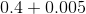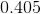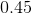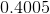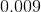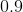Explanation:

In order to add the decimals, add placeholders to the decimal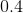.

Be careful not to add the wrong digits!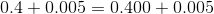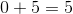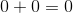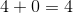Combine the numbers and put a decimal before the tenths place.

The correct answer is:### Example Question #3 : How To Add Decimals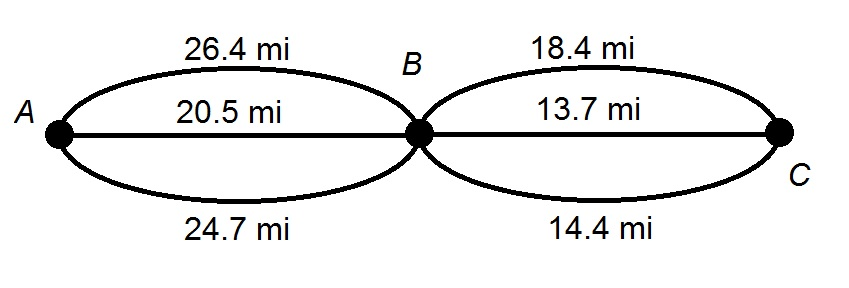A family is taking a trip from Town A to Town B, then to Town C. Above is a diagram of the routes available to them.

Give the range for the driving distancefor the trip.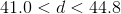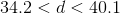None of the other choices gives the correct response.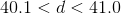Explanation:

Each route includes one path from Point A to Point B and one path from Point B o Point C.

The shortest possible drive is the sum of the shortest paths for each leg of the trip: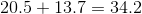The longest possible drive is the sum of the longest paths for each leg of the trip: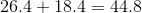The correct response is that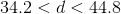.

### Example Question #4 : How To Add DecimalsAbove is a simplified map of the routes from Town A to Town B, and the routes from Town B to Town C.

A family wants to travel from Town A to Town C by way of Town B, then back to Town A by way of Town B. Since all routes are scenic, the family does not want to take any route twice.

Give the range for the distancein miles that the family will travel.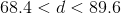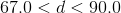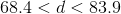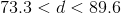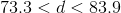Explanation:

The family's trip will be designed so that the family will take two different routes of the three that connect Town A and Town B, and two different routes of the three that connect Town B and Town C.

The minimum distance that the family will travel is therefore the sum of the lengths of the two shortest routes from Town A to Town B, and those of the two shortest routes from Town B to Town C: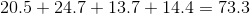miles

The maximum distance that the family will travel is, similarly, the sum of the lengths of the two longest routes from Town A to Town B, and those of the two longest routes from Town B to Town C: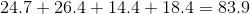miles

The correct choice is therefore.

### Example Question #5 : How To Add DecimalsA family is taking a trip from Town A to Town B, then to Town C. Above is a diagram of the routes available to them. How many routes will only require them to drive 40 miles or fewer:

Four

Three

Two

Six

Five

Five

Explanation:

There are three routes from Point A to Point B, and three from Point B to Point C, for a total of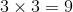routes total. The total distance traveled is the distance of one of the first three routes added to that of one of the last three; we can take all nine possibilities and add the distances: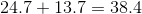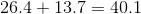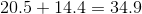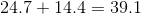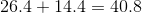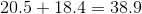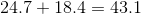Five of these routes require driving a distance 40 miles or fewer.